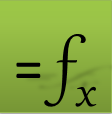# =QUADXY(x, y, [options])

QUADXY is a powerful function which employs splines to compute the integral of a function defined by a set of (x,y) data points.

With options, you can elect to weigh the data points, use exact or smooth least square fit, as well as specify end points slopes if known.

QUADXY automatically sorts your data points and averages the y values if your data set contains duplicate x points.

### Required Inputs

x a vector of the points x-coordinates.

y corresponding vector of the points y-values.

### Optional Inputs

ctrl a set of key/value pairs for algorithmic control as detailed below.

Description of key/value pairs for algorithmic control

 Key ORDER Admissible Values (Integer) 1 or 3 (linear or cubic) Default Value 3
 Keys ISLOPE, ESLOPE Admissible Values real number Default Value Unconstrained Remarks If defined, the spline curve first derivative will match the supplied value. Cannot be imposed with a linear spline or with PERIOIDC option enabled.
 Key PERIODIC Admissible Values (Boolean) True or False Default Value False Remarks The y-values at the end points must be equal if PERIOIDC is set to True. ISLOPE, ESLOPE constraints are ignored
 Key SFACTOR Admissible Values (real) ≥ 0 Default Value 0 Remarks A zero value will compute an exact interpolating spline passing through the data points. A large value will compute a least-squares smooth fitting curve. The smoothing factor has a significant effect on the computed integral value.

limits integration lower and upper limits if different from the data set end points. Must be within data set. Passing a single value defines the lower limit only. passing a vector of 2 values defines both limits.

w strictly-positive corresponding set of weights for the (x,y) data points. Default value is unity.

In this example we sample the function , then integrate the sampled data using QUADXY and compare the result to the exact value:

### Solution

Define a vector for the x values in range A1:A21 from 1 to 2 in increments of 0.05. Using AutoFill, generate the corresponding y values as shown in Table 1 from the formula =(2*A1^5 -A1+3)/A1^2 in B1.

 A B 1 1 4 2 1.05 4.083957 3 1.1 4.232248 4 1.15 4.440616 5 1.2 4.706 6 1.25 5.02625 7 1.3 5.399917 8 1.35 5.8261 9 1.4 6.304327 10 1.45 6.834468 11 1.5 7.416667 12 1.55 8.051288 13 1.6 8.738875 14 1.65 9.480118 15 1.7 10.27583 16 1.75 11.12691 17 1.8 12.03437 18 1.85 12.99926 19 1.9 14.02271 20 1.95 15.10588 21 2 16.25

Table 2 shows the numerical integral value computed by QUADXY formula as well as the analytical exact integral value.

 C 1 8.306853473 2 8.306852819

QUADXY computes the integral by fitting a linear or cubic spline model to the data.

Question or Comment? Email us:
support @ excel-works.comExceLab: Transforming Excel into a Calculus Power House

ExceLab functions and methods are protected by USA Patents 10628634, 10114812, 9892108 and 9286286.